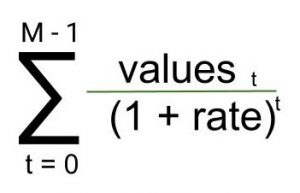# numpy.npv() in Python

numpy.npv(rate, value) : This financial function helps user to calculate the NPV(Net Present Value) of a cash flow series.

Parameters :

```rate  : [scalar] Rate of discount
value : [array_like, shape(M,)] value of
cash flows time series. The (fixed) time interval
between cash flow “events” must be the same as that
for given rate is given. By convention, investments
or “deposits” are -ve, income or “withdrawals” are +ve
```

Return :

```present value as per given parameters.
```

Equation being solved :Code 1 : Working

 `## Python program explaining pv() function ` `import` `numpy as np ` ` `  `#            rate            values      ` `a ``=`  `np.npv(``0.281``,[``-``100``, ``19``, ``49``, ``58``, ``200``]) ` `print``(``"Net Present Value(npv) : "``, a) `

Output :

```Net Present Value(npv) :  46.5579792365
```
My Personal Notes arrow_drop_upIf you like GeeksforGeeks and would like to contribute, you can also write an article using contribute.geeksforgeeks.org or mail your article to contribute@geeksforgeeks.org. See your article appearing on the GeeksforGeeks main page and help other Geeks.

Please Improve this article if you find anything incorrect by clicking on the "Improve Article" button below.

Article Tags :

1

Please write to us at contribute@geeksforgeeks.org to report any issue with the above content.